##### GRE: 1,001 Practice Questions For DummiesThe geometry problems on the GRE Math test may include basic shapes and 3-dimensional shapes. If you're fresh out of math class, you'll probably be fine going through these quickly.

If it's been a few years since you've done any geometry, you may want to work these questions carefully. Students who take the GRE tend to make the following mistakes:

• Mistakes in interpreting the question, such as using the wrong line or angle measures

• Mistakes in working the problem, such as applying the wrong formula

• Not reading the question carefully, and missing what they must actually look for

The following practice questions will test your skills at dealing with both a simple shape and a 3-dimensional shape.

## Practice questions

1. Find the area.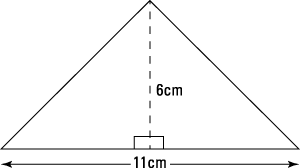A. 17 cm

B. 23 cm

C. 33 cm

D. 60 cm

E. 66 cm

2. Based on this diagram,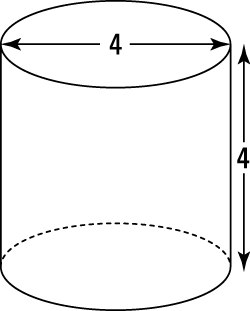calculate the total surface area of the cylinder.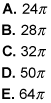1. C.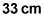The area is found by using the formula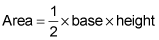Substitute the values from the diagram into the formula: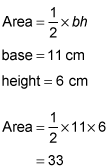The area is 33 centimeters, Choice (C).

2. A.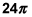Use the formula for surface area of a cylinder: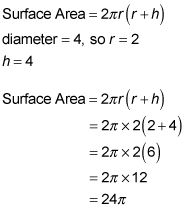The correct answer is Choice (A).